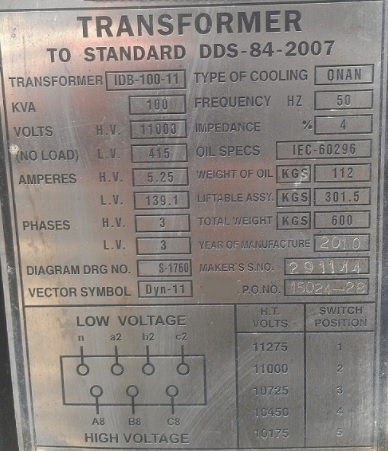# How to Calculate the Required KVA Rating for three Phase Transformers?

### A transformer is used to convert the incoming voltage at the location to the correct voltage for the electrical device (the submersible motor in case of ESP). Transformer selection is based on mainly 4 parameters:

• Power rating in KVA (Kilo Volt Amperes),
• Primary voltage,
• Secondary voltage,
• Tap arrangement.

In this post, we’ll detail how to calculate the required KVA for a 3 phase transformer. Actually, The calculation of KVA capacity for a Three Phase Transformer is based on Winding Voltage and Amperage information. The simple formula to calculate the rating of three phase Transformers is:

## KVA = (√3. V x I) /1000

Where: V is the voltage (volts) and I is the current (amps).

### Example:

the following figure presents a nameplate of a three phase transformer. It’s rating is 100 KVA. Using the aforementioned formula, let’s confirm this rating:

• Primary Voltages or High Voltages (H.V) is 11000 volts.
• Primary Current (current on High Voltage side) is 5.25 amps.
• KVA = (√3. V x I) /1000= (1.732 × 11000 × 5.25)/1000=100 KVA

Similarly:

• Secondary voltages or Low Voltages (L.V) is 415 volts.
• Secondary Current (Current on Low voltages side) is 139.1 amps.
• KVA = (√3. V x I) /1000= (1.732 × 415 × 139.1)/1000=100 KVA

This leads to the fact that the KVA rating of a transformer is the same for either the primary or secondary.

(KVA)in = (KVA)outPS: For more information related to transformer: how it works? key components? types of transformers?, refer to the post titled: ” Introduction to transformer: How it works? “.